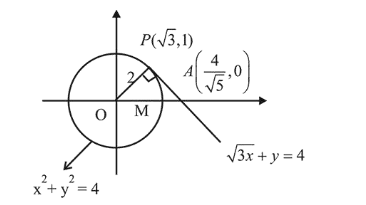# The tangent and the normal linesQuestion:

The tangent and the normal lines at the point $(\sqrt{3}, 1)$ to the circle $x^{2}+y^{2}=4$ and the $x$-axis form a triangle. The area of this triangle (in square units) is :

1. (1) $\frac{4}{\sqrt{3}}$

2. (2) $\frac{1}{3}$

3. (3) $\frac{2}{\sqrt{3}}$

4. (4) $\frac{1}{\sqrt{3}}$

Correct Option: , 3

Solution:

Equation of tangent to circle at point $(\sqrt{3}, 1)$ is

$\sqrt{3} x+y=4$$\therefore$ coordinates of the point $\quad\left(\frac{}{\sqrt{ }}, 0\right)$

Area $=\frac{1}{2} \times O A \times P M=\frac{1}{2} \times \frac{4}{\sqrt{3}} \times 1=\frac{2}{\sqrt{3}}$ sq. units